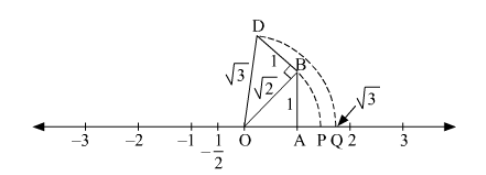# Locate 3–√ on the number line.

Question:

Locate $\sqrt{3}$ on the number line.

Solution:To represent $\sqrt{3}$ on the number line, follow the following steps of construction:

(i) Mark points 0 and 1 as $\mathrm{O}$ and $\mathrm{A}$, respectively.

(ii) At point $A$, draw $A B \perp O A$ such that $A B=1$ units.

(iii) Join OB.

(iv) At point $B$, draw $D B \perp O A$ such that $D B=1$ units.

(v) Join OD.

(vi) With $O$ as centre and radius $O D$, draw an arc intersecting the number line at point $Q$.

Thus, point $Q$ represents $\sqrt{3}$ on the number line.

Justification:

In right Unexpected text node: '∆'OAB,Using Pythagoras theorem,

$\mathrm{OB}=\sqrt{\mathrm{OA}^{2}+\mathrm{AB}^{2}}$

$=\sqrt{1^{2}+1^{2}}$

$=\sqrt{1+1}$

$=\sqrt{2}$

Again, in right $\triangle O D B$,

Using Pythagoras theorem,

$\mathrm{OD}=\sqrt{\mathrm{OB}^{2}+\mathrm{DB}^{2}}$

$=\sqrt{(\sqrt{2})^{2}+1^{2}}$

$=\sqrt{2+1}$

$=\sqrt{3}$